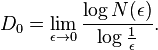# Help with fractal dimensions!

First post!

I'm investigating chaos in non linear coupled spring oscillators. After generating a poincare' map of said system i wanted to see if the map was fractal. i proceeded to use a box counting method in order to calculate a fractal dimension.

I generated a plot of log(number of occupied boxes) versus log(total boxes) and the slope should be the fractal dimension, i think. However my slope was approximately .65

Is this acceptable? I've only seen fractal dimensions of values between 1 and 2. Which leads me to believe i've done something wrong. any help?

also if i posted in the wrong place i apologize in advance.

Pythagorean
Gold Member
should be more than 1. Dont you need to guarantee that the structures in each box are self-similar?

Pythagorean
Gold Member
I think this is more appropriate for your data:where e is the s box size